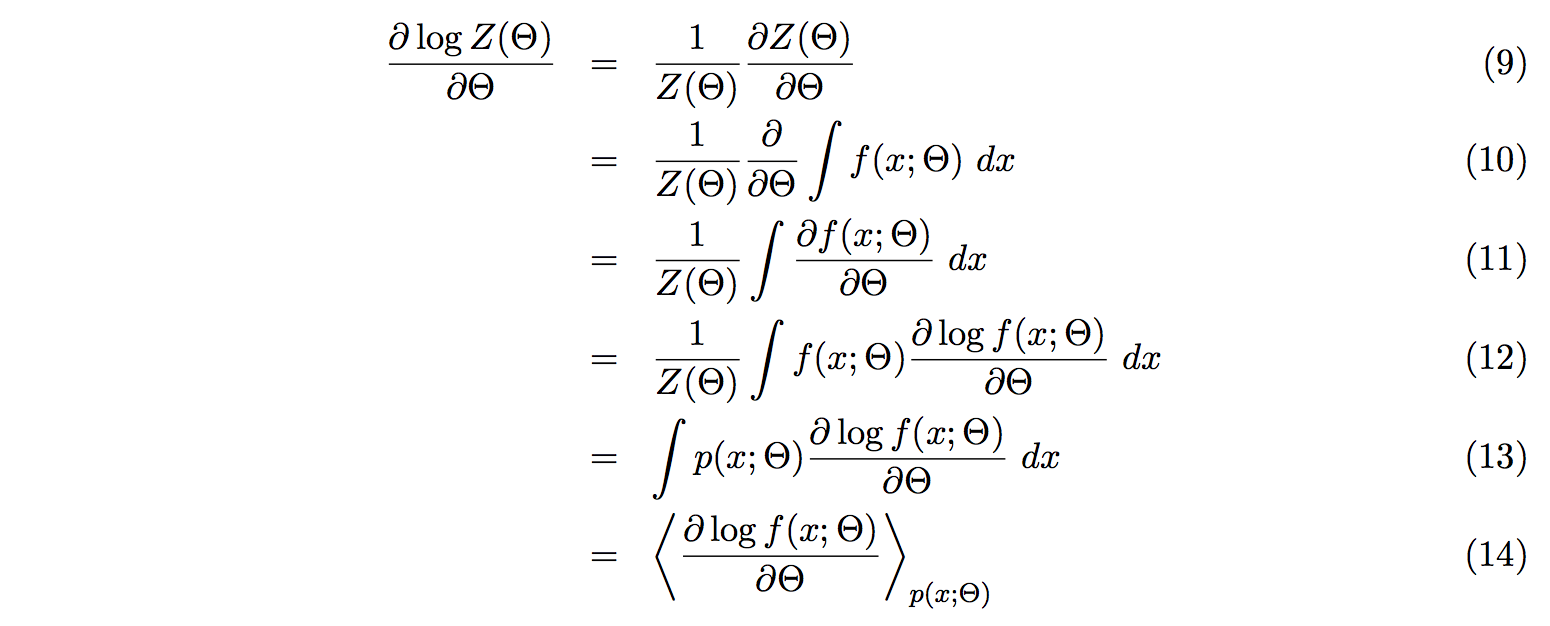For a long time, I did not get how contrastive divergence (CD) works. I was stumped by the bracket notation, and by “maximizing the log probability of the data”.

Local copy here (in case website is down)

The only math needed is integrals, partial derivatives, sum, products, and the derivative of the log of an arbitrary function. `(log u)' = u' / u`.

Just so the math fits on one screen, I’ll put the equations below.

`x` is a data point. `f(x; Θ)` is our function. `Θ` is a vector of model parameters.`Z(Θ)` is the partition function.We learn our model parameters, `Θ`, by maximizing the probability of a training set of data, `X = x1,..,K`, given asWhich is the same as minimizing the energy `E(X; Θ)`We derive the energy with respect to the model parameters, `Θ`

Quick explanation for (7): for first bit `d log Z(Θ)`, we just put the `d/dΘ` on top of it. For the second bit, we put the `d/dΘ` inside the sum.

Quick explanation for (8): The sum is rewritten with the bracket notation.Here we calculate the first bit of the energy derivative of (7)

(9): We use `(log u)' = u' / u`

(9 -> 10): We use the definition of Z. See (2)

(10-> 11): Easy

(11 -> 12): We use `(log u)' = u' / u` again.

(12 -> 13): We use the definition of p. See (1)